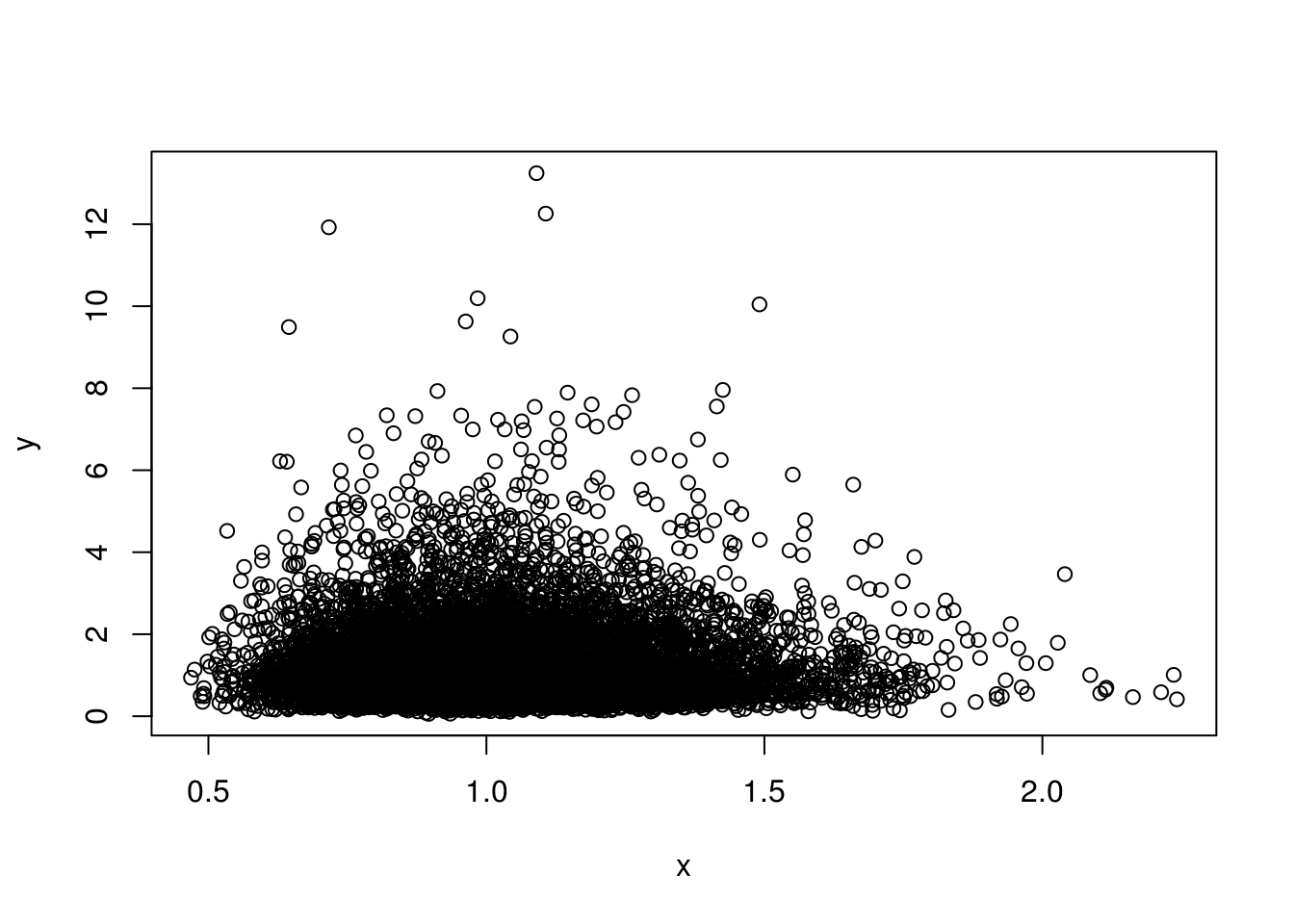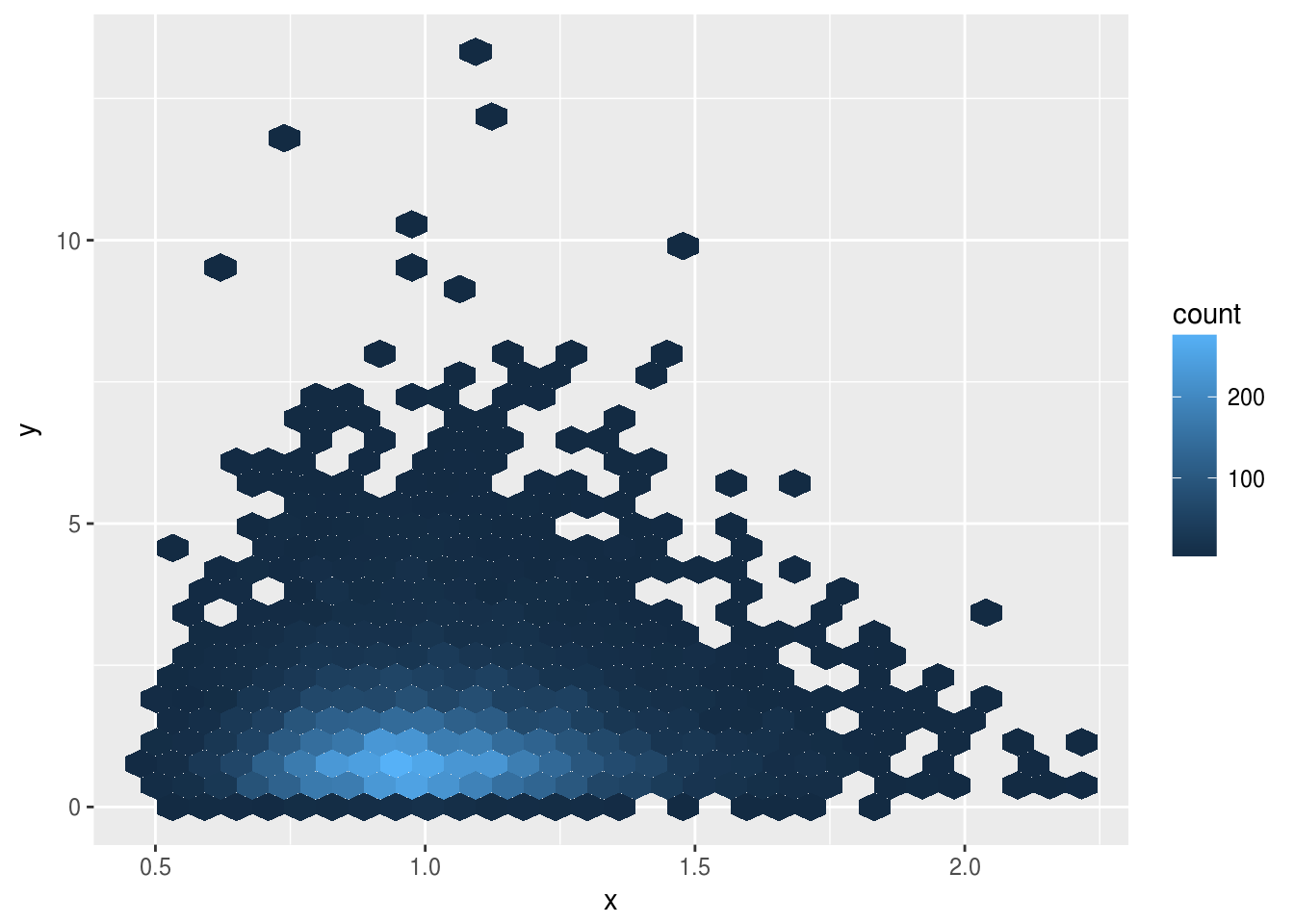``````library(tidyverse)
library(viridis)
library(RANN)``````

## The problem of scatterplots with too many points

Consider some 2D data.

``````set.seed(1234)

n <- 10000
x <- 1.25^rnorm(n)
y <- 2^rnorm(n)

plot(x,y)``````It isn’t clear what is going on in the solid black part. Binning the data may help.

``data_frame(x=x,y=y) %>% ggplot(aes(x=x,y=y)) + geom_hex()``Binning the data shows there is a higher density section. Beyond this it is hard to read anything from this type of density plot. What fraction of points are in the high density section? Hard to say. With a 1D histogram, we could look at the area under the plot. Here we can’t easily estimate the volume when one of the dimensions is color.

We have also obscured that this is a scatterplot. A multitude of sins along these lines have been devised, which I shan’t enumerate.

## My solution

Attempting to channel the spirit of John Tukey, my proposal is to divide the points into four equally sized groups by density.

``````scatter_plot <- function(x,y,k=min(25,length(x)),n=4) {
df <- data.frame(x=x,y=y)

# Estimate density by k-nearest neighbours
# (a kernel density estimate might be used instead)
result <- nn2(scale(df), k=k)
df\$kdist <- result\$nn.dists[,k]

# Divide points into n groups by density
df\$group <-
ceiling(rank(-df\$kdist, ties.method="random") *(n/nrow(df))) %>%
factor(levels=seq_len(n))

arrange(df, -kdist) %>%
ggplot(aes(x=x,y=y,color=group)) +
geom_point(size=2, col="black") +
geom_point(size=1.75) +
scale_color_viridis(discrete=TRUE, labels=rep(paste0("1/",n),n)) +
labs(x="",y="",color=paste0("Fraction of\n",length(x)," points"))
}

scatter_plot(x,y)``````